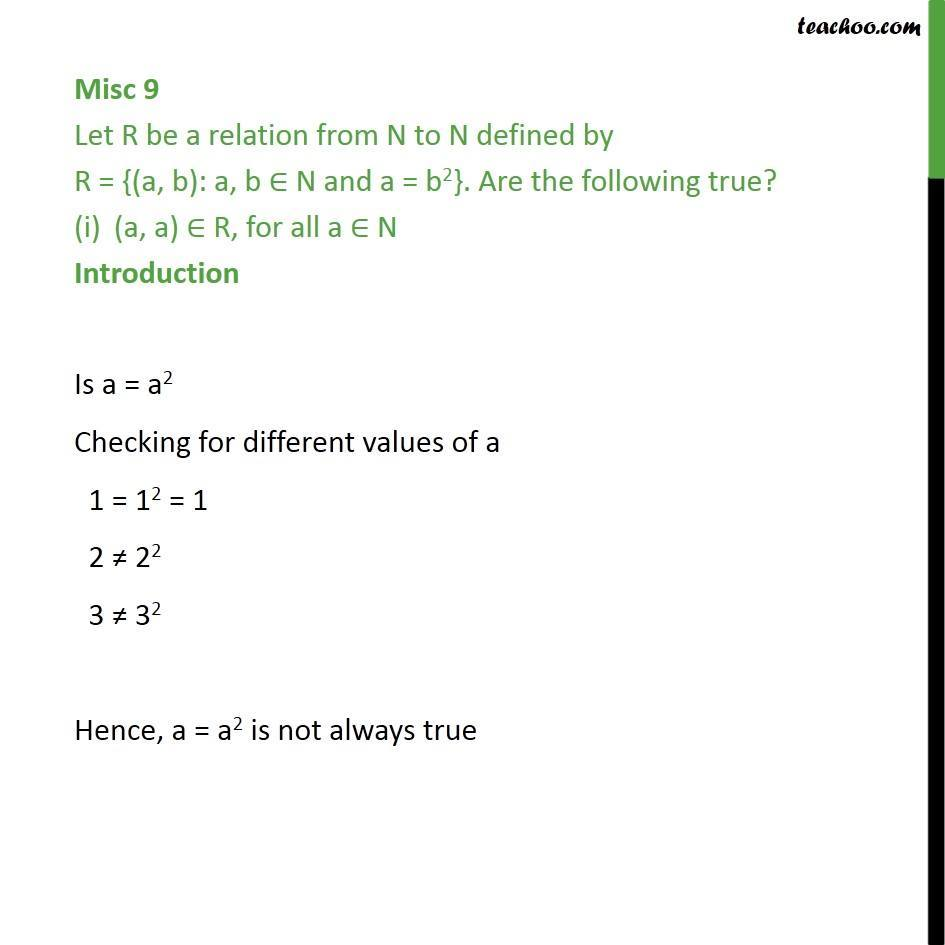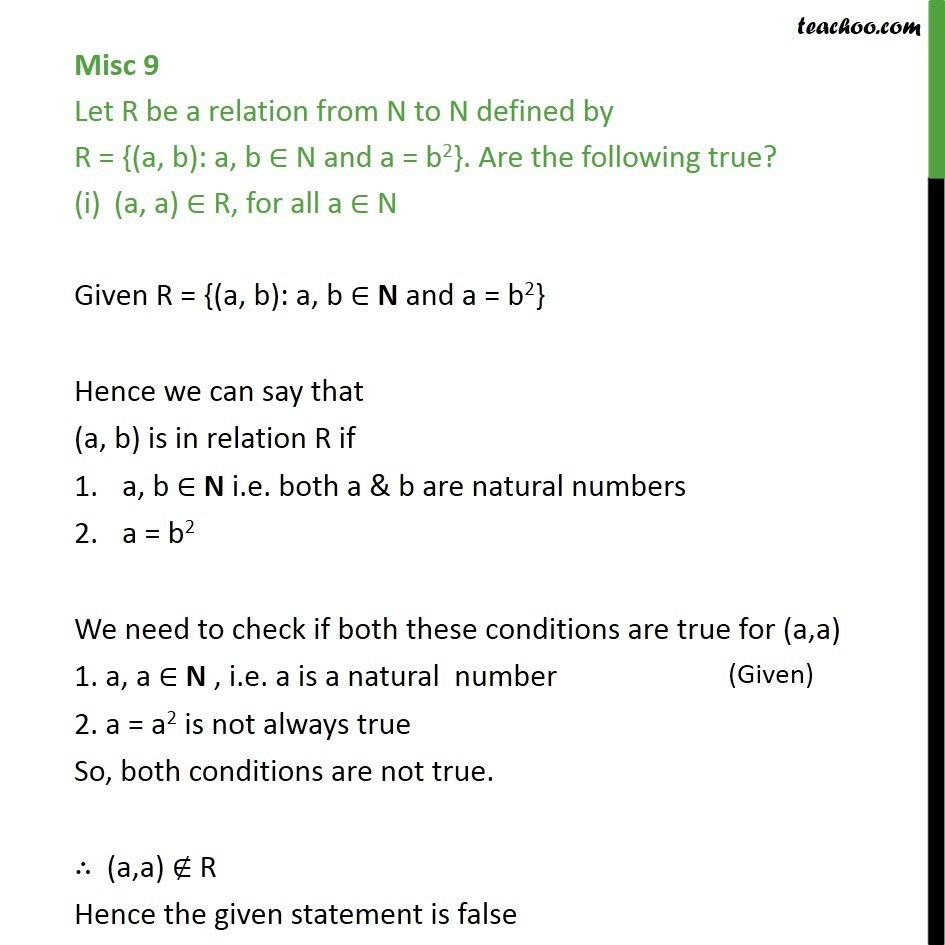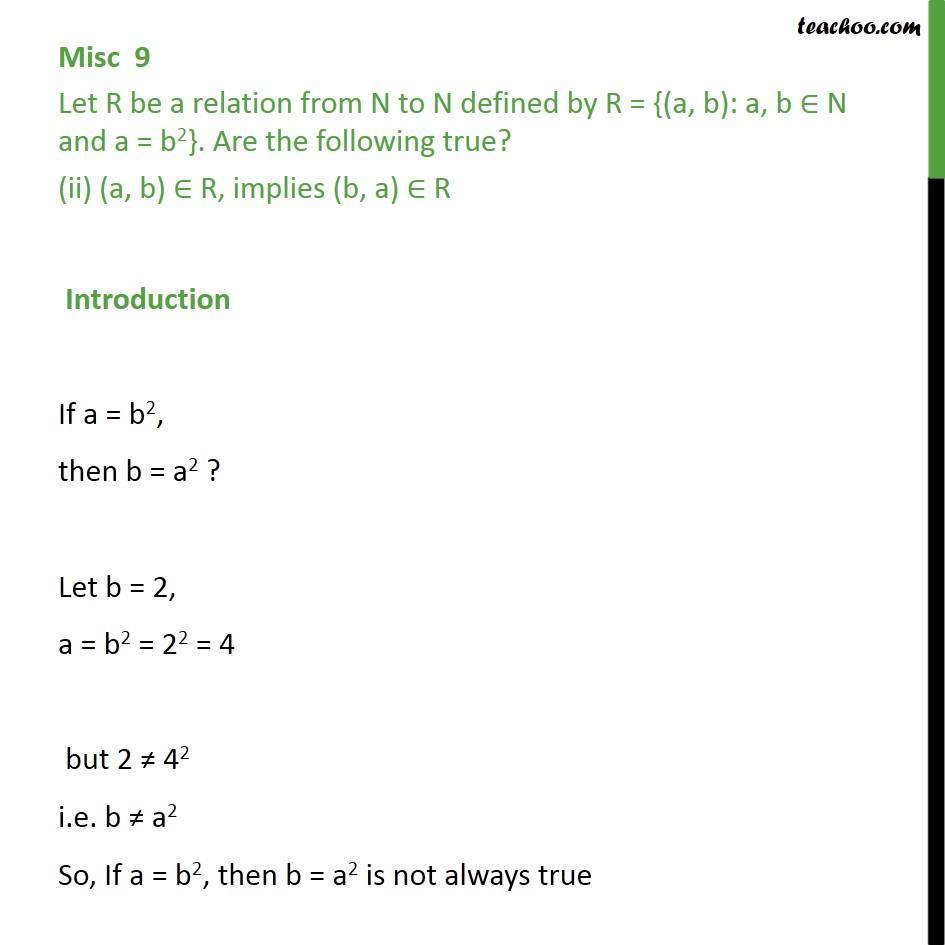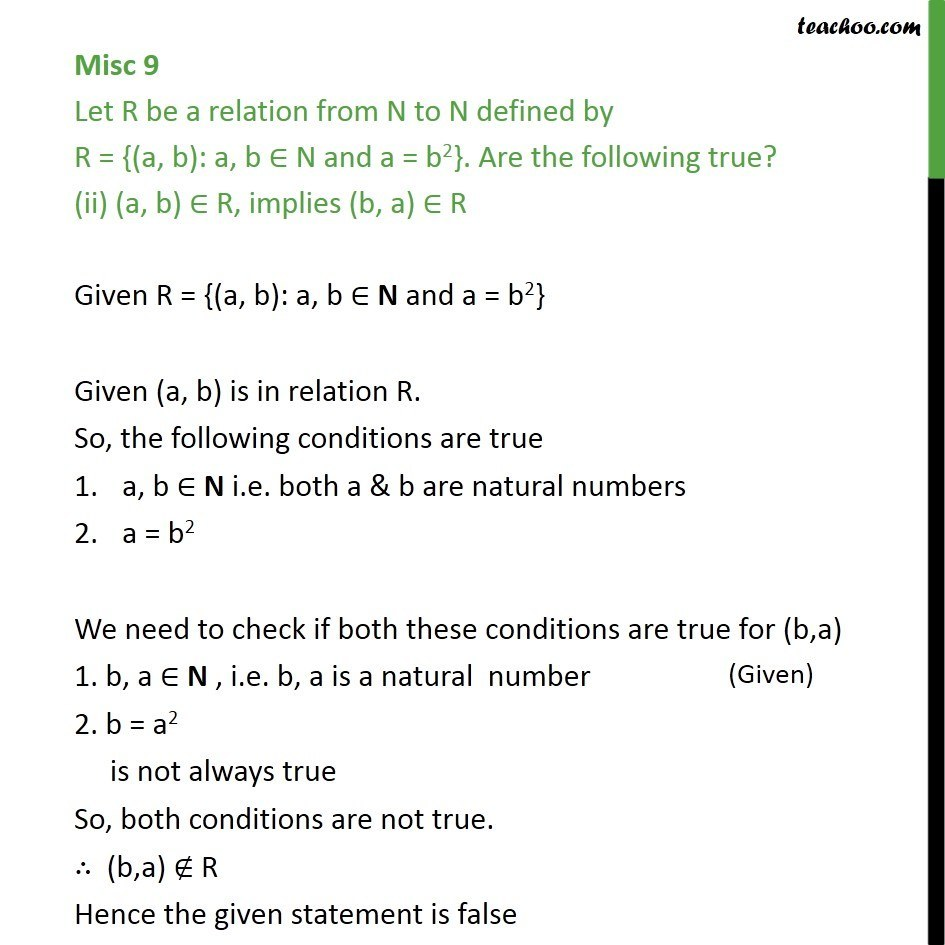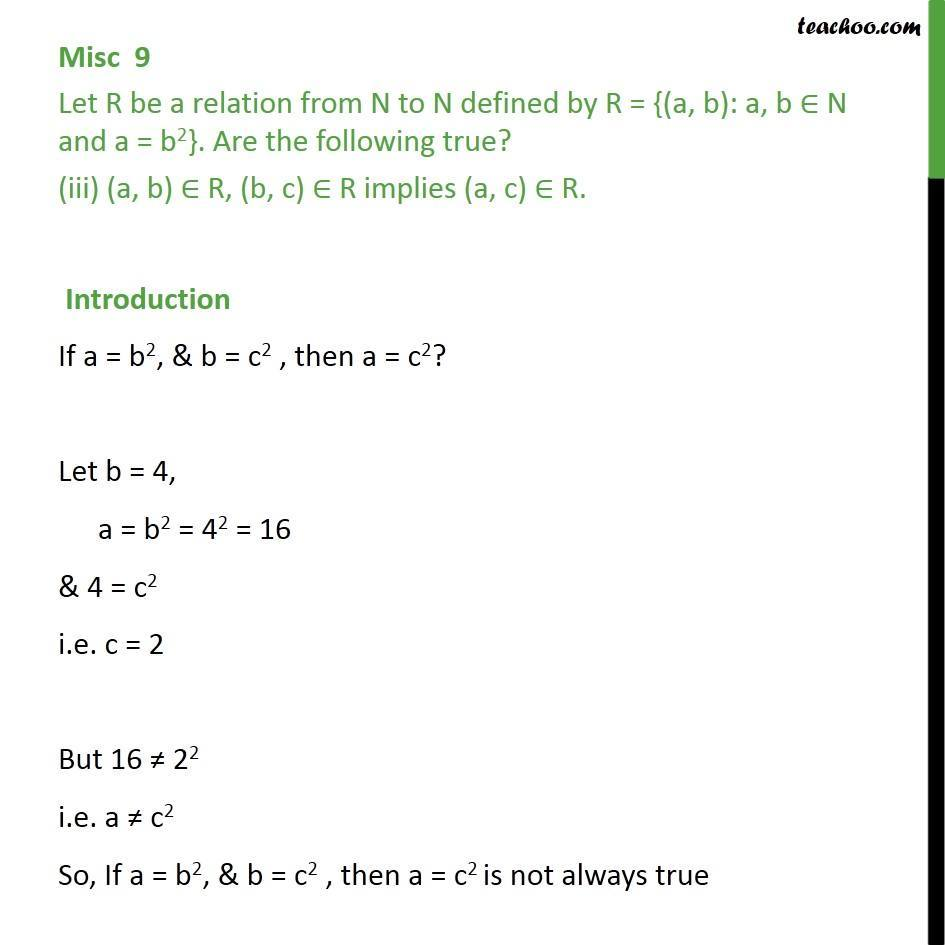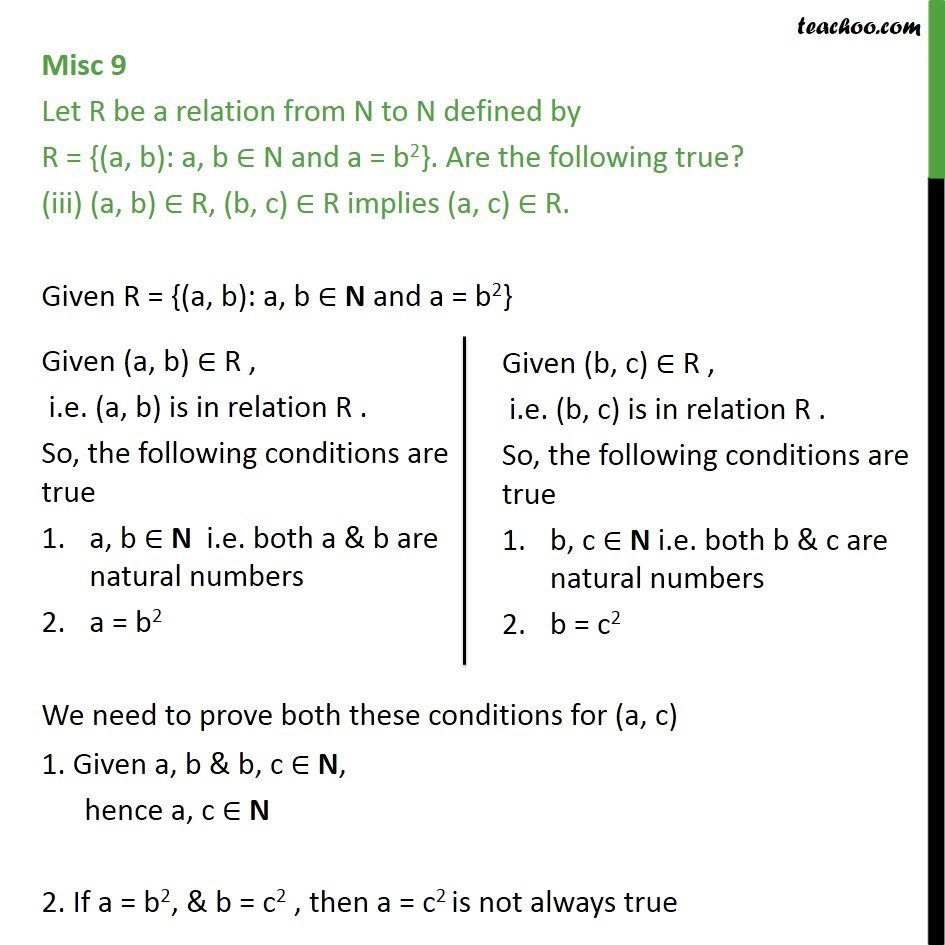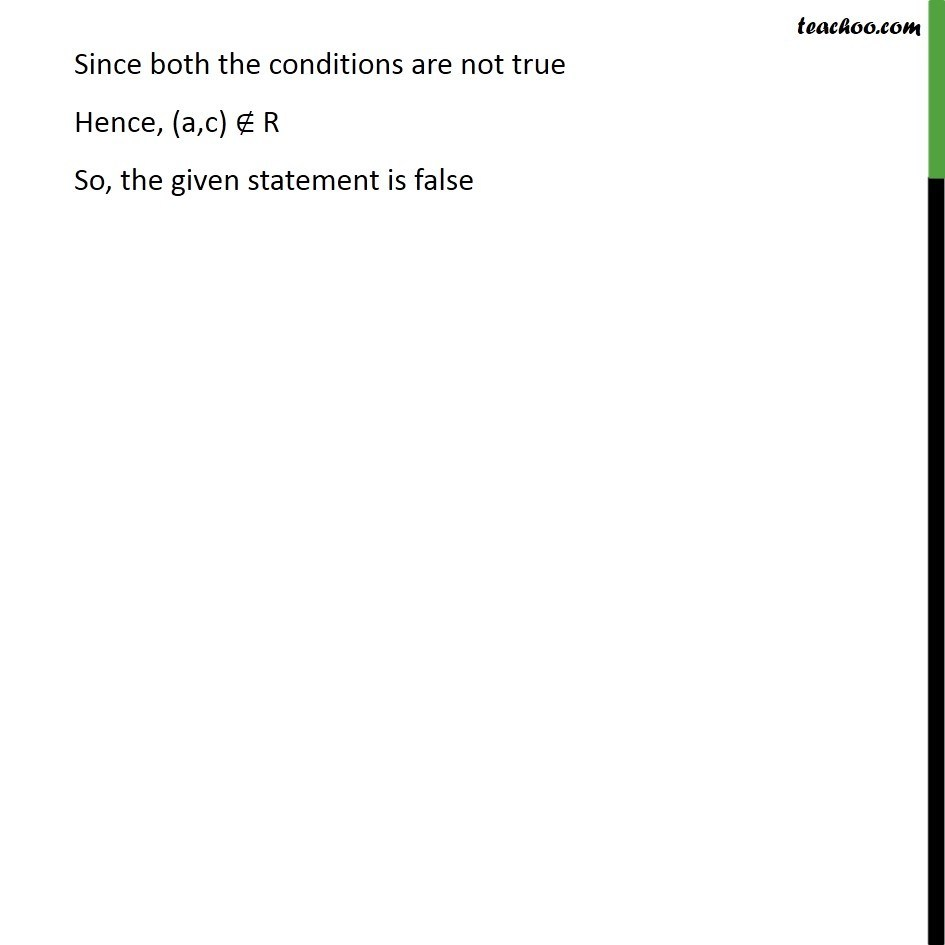1. Chapter 2 Class 11 Relations and Functions
2. Concept wise
3. Relations - Definition

Transcript

Misc 9 Let R be a relation from N to N defined by R = {(a, b): a, b N and a = b2}. Are the following true? (a, a) R, for all a N Introduction Is a = a2 Checking for different values of a 1 = 12 = 1 2 22 3 32 Hence, a = a2 is not always true Misc 9 Let R be a relation from N to N defined by R = {(a, b): a, b N and a = b2}. Are the following true? (a, a) R, for all a N Given R = {(a, b): a, b N and a = b2} Hence we can say that (a, b) is in relation R if a, b N i.e. both a & b are natural numbers a = b2 We need to check if both these conditions are true for (a,a) 1. a, a N , i.e. a is a natural number 2. a = a2 is not always true So, both conditions are not true. (a,a) R Hence the given statement is false Misc 9 Let R be a relation from N to N defined by R = {(a, b): a, b N and a = b2}. Are the following true? (ii) (a, b) R, implies (b, a) R Introduction If a = b2, then b = a2 ? Let b = 2, a = b2 = 22 = 4 but 2 42 i.e. b a2 So, If a = b2, then b = a2 is not always true Misc 9 Let R be a relation from N to N defined by R = {(a, b): a, b N and a = b2}. Are the following true? (ii) (a, b) R, implies (b, a) R Given R = {(a, b): a, b N and a = b2} Given (a, b) is in relation R. So, the following conditions are true a, b N i.e. both a & b are natural numbers a = b2 We need to check if both these conditions are true for (b,a) 1. b, a N , i.e. b, a is a natural number 2. b = a2 is not always true So, both conditions are not true. (b,a) R Hence the given statement is false Misc 9 Let R be a relation from N to N defined by R = {(a, b): a, b N and a = b2}. Are the following true? (iii) (a, b) R, (b, c) R implies (a, c) R. Introduction If a = b2, & b = c2 , then a = c2? Let b = 4, a = b2 = 42 = 16 & 4 = c2 i.e. c = 2 But 16 22 i.e. a c2 So, If a = b2, & b = c2 , then a = c2 is not always true Misc 9 Let R be a relation from N to N defined by R = {(a, b): a, b N and a = b2}. Are the following true? (iii) (a, b) R, (b, c) R implies (a, c) R. Given R = {(a, b): a, b N and a = b2} We need to prove both these conditions for (a, c) 1. Given a, b & b, c N, hence a, c N 2. If a = b2, & b = c2 , then a = c2 is not always true Since both the conditions are not true Hence, (a,c) R So, the given statement is false

Relations - Definition